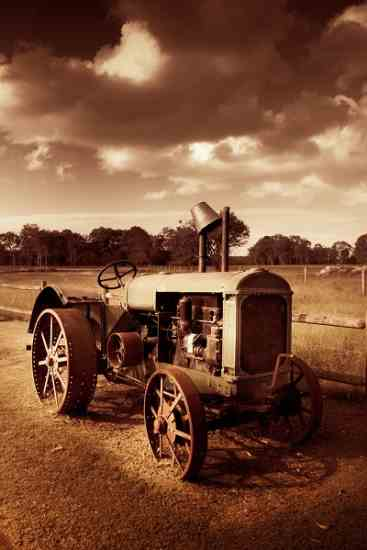# Formula For Determining Horsepower

By StaffHere is an easy formula for determining the horsepower
of most any gas engine and any number of cylinders. We found this
article in the January 1926 issue of American Thresherman Magazine.
Taking the bore and stroke of several different engines we have
here and figuring it all out, we find that it checks out mighty
close too.

Take the diameter or bore and multiply it by itself on a four
inch bore this gives sixteen. Now multiply sixteen by the stroke,
say five inches, giving eighty. Next multiply eighty by the R.P.M.,
and at say 1,000 R.P.M. this gives 80,000. Now divide by 13,000 for
a four-cycle engine or by 10,000 for a two cycle engine. In this
case of the 4 x 5 at 1,000 R.P.M. we get practically six horsepower
or eight horsepower for a two cycle. For a four cylinder engine,
say, just calculate the one cylinder and multiply by four and it
will come out pretty close. Putting it in a formula it looks like
this:

H.P =

D2 x L x R.P.M. / 13,000 x  N (Numbers of
cylinders)

Remember to use 13,000 for a four cycle engine and 10,000 for a
two cycle engine.

• Published on May 1, 1966